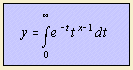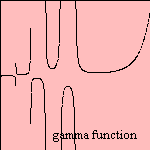# gamma function

## gammaThe gamma function Γ(x) can be seen as a generalization of the factorial: for a positive integer the function value of the gamma function is equal to the factorial.

Alternative definitions of the curve are defined by Bourbaki and Weierstrass (with infinite products).

The gamma function has some remarkable qualities: it has an infinite number of maxima and minima. And it can be proven that it cannot be the solution of a differential equation with algebraic coefficients.
The function plays a major role in difference equations, as the exponential functions has its role for differential equations. The curve can be found in a lot of mathematical problems.

The name and the notation of the function has been set by Legendre (1809). Because of the work of Euler on the curve the function is also called the Euler gamma function or the second Euler function.

On the internet approximations of gamma functions can be found, for instance on the site of Victor T. Toth.